# NameError: name 'plt' is not defined in Python [Solved]Last updated: Feb 1, 2023
3 min## #NameError: name 'plt' is not defined in Python

The Python "NameError: name 'plt' is not defined" occurs when we use the `pyplot` module without importing it first.

To solve the error, install `matplotlib` and import `plt` (`import matplotlib.pyplot as plt`) before using it.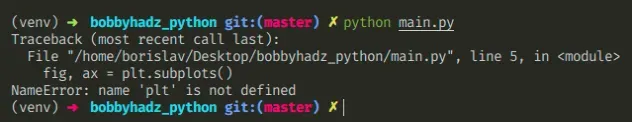Open your terminal in your project's root directory and install the matplotlib module.

shell
```Copied!```# 👇️ in a virtual environment or using Python 2
pip install matplotlib

# 👇️ for python 3 (could also be pip3.10 depending on your version)
pip3 install matplotlib
``````

If you get a permissions error on macOS or Linux, use the `sudo` prefix.

shell
```Copied!```# 👇️ if you get permissions error
sudo pip3 install matplotlib
``````

If you don't have pip in your PATH environment variable, use the `python -m pip` command.

shell
```Copied!```# 👇️ if you don't have pip in your PATH environment variable
python -m pip install matplotlib

# 👇️ for python 3 (could also be pip3.10 depending on your version)
python3 -m pip install matplotlib

# 👇️ alternative for Ubuntu/Debian
sudo apt-get install python3-matplotlib

# 👇️ alternative for CentOS
sudo yum install python3-matplotlib

# 👇️ alternative for Fedora
sudo yum install python3-matplotlib

# 👇️ for Anaconda
conda install -c conda-forge matplotlib

# 👇️ for Jupyter Notebook
!pip install matplotlib
``````

After you install the matplotlib module, make sure to import `pyplot` before using it.

main.py
```Copied!```# 👇️ import pyplot and alias it as plt
import matplotlib.pyplot as plt

fig, ax = plt.subplots()

print(fig)
print(ax)
``````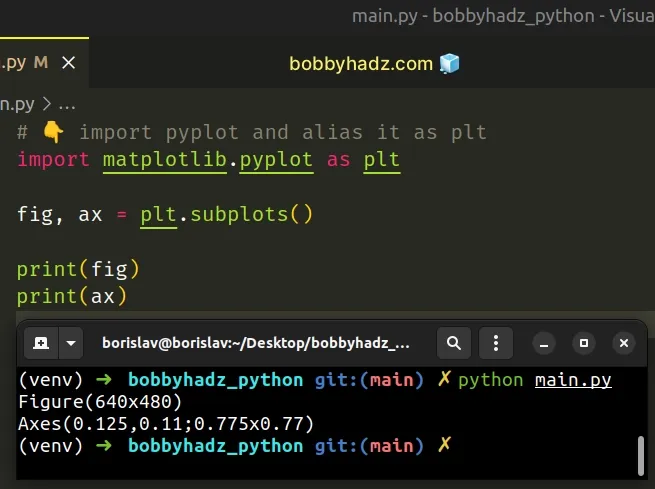We used an alias to import the `pyplot` module from `matplotlib` as `plt`.

We imported `matplotlib.pyplot` and aliased it to `plt`, so you would access any pyplot methods as `plt.plot()`, `plt.ylabel()`, `plt.show()`, etc.

Make sure you haven't misspelled the module you are importing because module names are case-sensitive.

You can also use `from` in your import statement when importing `pyplot` and aliasing it.

main.py
```Copied!```from matplotlib import pyplot as plt

fig, ax = plt.subplots()

print(fig)
print(ax)
``````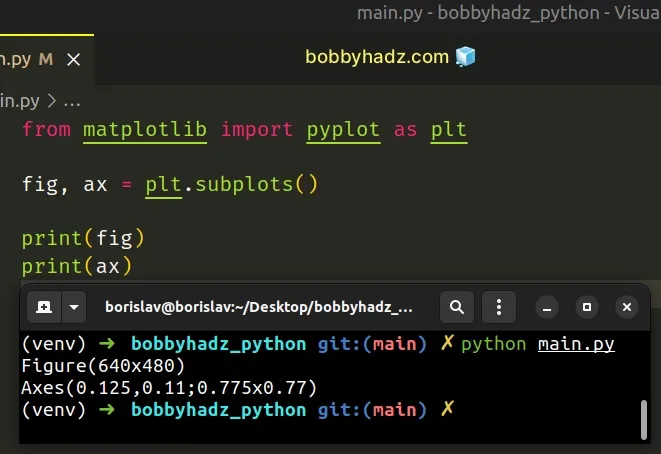The two code samples achieve the same result. They import `pyplot` from `matplotlib` and alias it to `plt`.

## #Make sure to not import the `matplotlib` module in a nested scope

Make sure you haven't placed your import statement in a nested scope, e.g. a function.

main.py
```Copied!```def example():
import matplotlib.pyplot as plt

fig, ax = plt.subplots()

print(fig)
print(ax)

# ⛔️ NameError: name 'plt' is not defined
fig, ax = plt.subplots()
``````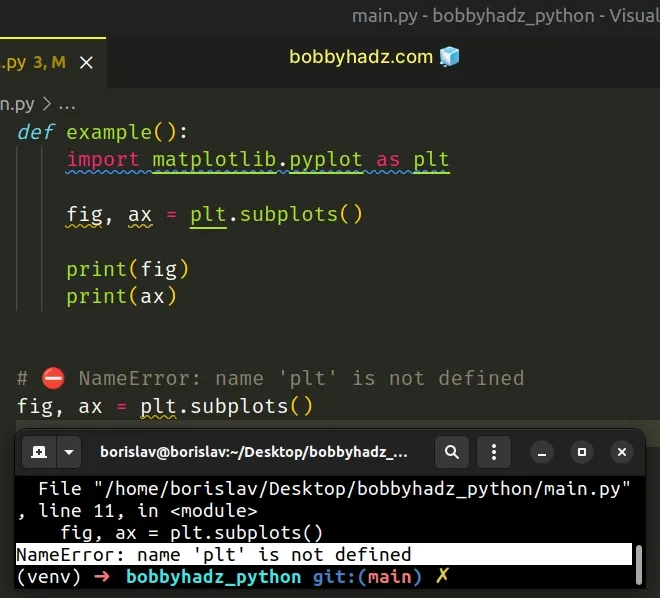We imported the `matplotlib` module in a function, so we aren't able to use it outside of the function.

Import the module at the top level to be able to use it throughout your code.

main.py
```Copied!```# ✅ moved import to top level
import matplotlib.pyplot as plt

def example():

fig, ax = plt.subplots()

print(fig)
print(ax)

fig, ax = plt.subplots()

print(fig)
print(ax)
``````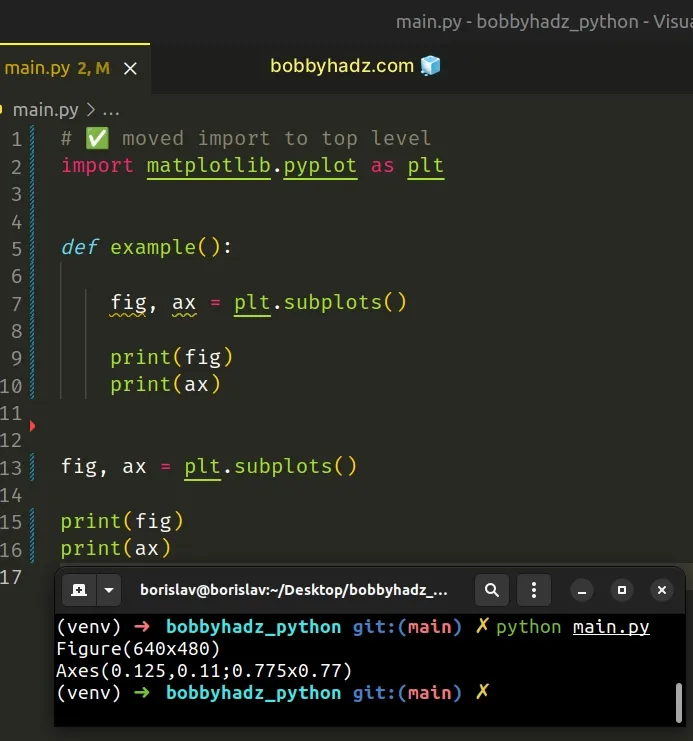The import statement for the `matplotlib` module has to come at the top of the file before any code that makes use of it.

## #Make sure to not import the `matplotlib` module in a try/except statement

You also should be importing the `matplotlib` module in a try/except statement.

main.py
```Copied!```try:
# 👉️ code here could raise an error

import matplotlib.pyplot as plt
fig, ax = plt.subplots()

print(fig)
print(ax)
except ImportError:
fig, ax = plt.subplots()

fig, ax = plt.subplots()
``````

The code sample works, however, if the code in the `try` statement raises an error, the `matplotlib` module won't get imported successfully.

This would cause the error because we are trying to access properties on the `matplotlib` module in the outer scope and the `except` block.

Instead, move the import statement to the top of the file.

main.py
```Copied!```# ✅ moved import to top of file
import matplotlib.pyplot as plt

try:
fig, ax = plt.subplots()

print(fig)
print(ax)
except ImportError:
fig, ax = plt.subplots()

fig, ax = plt.subplots()
``````

## #NameError: name 'matplotlib' is not defined in Python

The solution to the "NameError: name 'matplotlib' is not defined" is the same.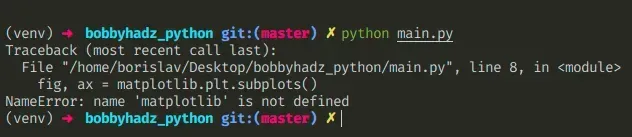After you install the `matplotlib` module, you have to import it before you are able to use it in your code.

main.py
```Copied!```# ✅ import matplotlib.pyplot and alias it to plt
import matplotlib.pyplot as plt

# ✅ import matplotlib.image and alias it to pmimg
import matplotlib.image as mpimg

fig, ax = plt.subplots()

print(fig)
print(ax)

print(img)
``````

We used aliases when importing from `matplotlib`.

We imported `matplotlib.pyplot` and aliased it to `plt`, so you would access any pyplot methods as `plt.plot()`, `plt.ylabel()`, `plt.show()`, etc.

Make sure you haven't misspelled the module you are importing because module names are case-sensitive.

Also, make sure you haven't imported from `matplotlib` in a nested scope, e.g. a function. Import the module at the top level to be able to use it throughout your code.

If you get the error ModuleNotFoundError: No module named 'matplotlib', click on the link and follow the instructions.

I wrote a book in which I share everything I know about how to become a better, more efficient programmer.You can use the search field on my Home Page to filter through all of my articles.